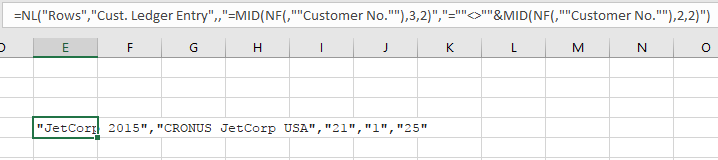The following statement returns rows where the Variety Code is equal to the 3rd and 4th characters of the Code.  What I really need is to return the rows where the Variety Code is NOT equal to the 3rd and 4th characters of the Code.  I'm struggling with getting the syntax correct.

=NL("Rows","Batch",,"=NF(,""Variety Code"")","=MID(NF(,""Code""),3,2)")

Thanks.

•Harry Lewis

Hi Yvonne -

Try this:

`=NL("Rows","Batch",,"=NF(,""Variety Code"")","=""<>""&MID(NF(,""Code""),3,2)")`

or, slightly simpler:

`=NL("Rows","Batch",,"Variety Code","=""<>""&MID(NF(,""Code""),3,2)")`

•Yvonne Jury

Ah, I get what we're trying to do here.  It's still not working though.  I have #VALUE in each of the cells, and when I run it I get an "Invalid filter "<>"." message.

•Harry Lewis

That would seem to indicate that the Code field can be blank.

If that is *not* the case, let try an experiment... are you able to enter this function:

`=NL("Rows","Cust. Ledger Entry",,"=MID(NF(,""Customer No.""),3,2)","=""<>""&MID(NF(,""Customer No.""),2,2)")`

(you don't have to run it... it doesn't really do anything useful).

Once entered, the worksheet should look something like this:•Yvonne Jury

Yes, that function works.

•Harry Lewis

What does this function return?

`=NL("Count","Batch",,"=MID(NF(,""Code""),3,2)","@@")`

•Yvonne Jury

There's 1.

•Harry Lewis

You could try this:

`=NL("Rows","Batch",,"Variety Code","=""<>@@""&MID(NF(,""Code""),3,2)")`

It might work.

•Yvonne Jury

That works!!  Thanks so much!!